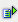The function sqrtpi (num) calculates the square root of a value pi.

Syntax: =sqrtpi()Example =sqrt(1) is 3.141593 = 1*pi =sqrt(2) is 4.442883 = sqrt(2)*pi =sqrt(3) is 5.441398 = sqrt(3)*pi =sqrt(4) is 6.283185 = sqrt(4)*pi